JEE  >  JEE Main 2019 April 10 Shift 1 Paper & Solutions

# JEE Main 2019 April 10 Shift 1 Paper & Solutions - JEE

Test Description

## 34 Questions MCQ Test JEE Main & Advanced Mock Test Series - JEE Main 2019 April 10 Shift 1 Paper & Solutions

JEE Main 2019 April 10 Shift 1 Paper & Solutions for JEE 2023 is part of JEE Main & Advanced Mock Test Series preparation. The JEE Main 2019 April 10 Shift 1 Paper & Solutions questions and answers have been prepared according to the JEE exam syllabus.The JEE Main 2019 April 10 Shift 1 Paper & Solutions MCQs are made for JEE 2023 Exam. Find important definitions, questions, notes, meanings, examples, exercises, MCQs and online tests for JEE Main 2019 April 10 Shift 1 Paper & Solutions below.
Solutions of JEE Main 2019 April 10 Shift 1 Paper & Solutions questions in English are available as part of our JEE Main & Advanced Mock Test Series for JEE & JEE Main 2019 April 10 Shift 1 Paper & Solutions solutions in Hindi for JEE Main & Advanced Mock Test Series course. Download more important topics, notes, lectures and mock test series for JEE Exam by signing up for free. Attempt JEE Main 2019 April 10 Shift 1 Paper & Solutions | 34 questions in 180 minutes | Mock test for JEE preparation | Free important questions MCQ to study JEE Main & Advanced Mock Test Series for JEE Exam | Download free PDF with solutions
 1 Crore+ students have signed up on EduRev. Have you?
JEE Main 2019 April 10 Shift 1 Paper & Solutions - Question 1

### A particle of mass m is moving along a trajectory given by x = x0 + a cosω1t. y = y0 + b sinω2t The torque,acting on the particle about the origin, at t=0 is :

Detailed Solution for JEE Main 2019 April 10 Shift 1 Paper & Solutions - Question 1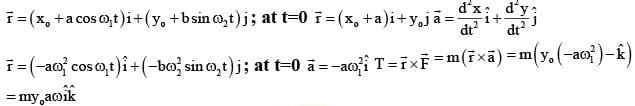JEE Main 2019 April 10 Shift 1 Paper & Solutions - Question 2

### A stationary source emits sound waves of frequency 500Hz. Two observers moving along a line passing through the source detect sound to be of frequencies 480 Hz ad 530Hz. Their respective speeds are,in ms-1 (given speed of sound =300 m/s)

Detailed Solution for JEE Main 2019 April 10 Shift 1 Paper & Solutions - Question 2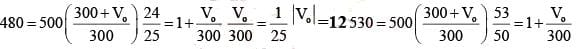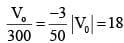Hence Velocities -12,18

JEE Main 2019 April 10 Shift 1 Paper & Solutions - Question 3

### n moles of an ideal gas with constant volume heat capacity Cv undergo an isobraric expansion by certain volume . The ratio of the work done in the process, to the heat supplied is :

Detailed Solution for JEE Main 2019 April 10 Shift 1 Paper & Solutions - Question 3

n=moles
cv=neat capacity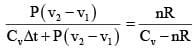JEE Main 2019 April 10 Shift 1 Paper & Solutions - Question 4

One Plano-convex and one plane of-concave lens of same radius of Curvature ‘R’ but of different materials are joined side by side as shown in the figure . If refractive index of the material of 1 is µ1 and that of 2 is µ2 ,then the focal length of the combination is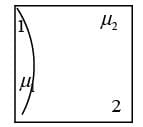Detailed Solution for JEE Main 2019 April 10 Shift 1 Paper & Solutions - Question 4

Diagram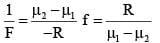JEE Main 2019 April 10 Shift 1 Paper & Solutions - Question 5

n a photoelectric effect experiment the threshold wavelength of the is 380nm. If the wavelength of indent is 260nm, the  maximum kinetic energy of emitted electrons will be: given E{in ev}= 1237/λ (innm)

Detailed Solution for JEE Main 2019 April 10 Shift 1 Paper & Solutions - Question 5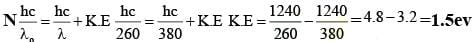JEE Main 2019 April 10 Shift 1 Paper & Solutions - Question 6

The electric field of a plane electromagnetic wave is given by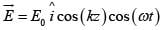The corresponding magnetic field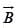is then given by :

Detailed Solution for JEE Main 2019 April 10 Shift 1 Paper & Solutions - Question 6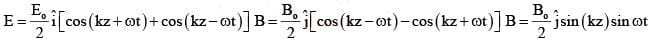JEE Main 2019 April 10 Shift 1 Paper & Solutions - Question 7

A transformer consisting of 300 turns in the primary and 150 turns in the secondary gives output power of 2.2kw. if the current in the secondary coil is 10A, then the input voltage and current in the primary Coil are:

Detailed Solution for JEE Main 2019 April 10 Shift 1 Paper & Solutions - Question 7

Np=300 Ns=150 V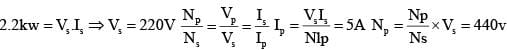JEE Main 2019 April 10 Shift 1 Paper & Solutions - Question 8

A cylinder with fixed capacity of 67.2lit contains helium gas at STP. The amount of heat needed to raise the temperature of the gas by 200C is : [Given that  R=8.31J mol -1k -1 ]

Detailed Solution for JEE Main 2019 April 10 Shift 1 Paper & Solutions - Question 8

67.2 A7STP n=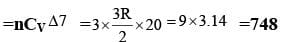JEE Main 2019 April 10 Shift 1 Paper & Solutions - Question 9

Two radioactive materials A and B have decay constants 10λ and λ,respectively . If initially they have the same number of nuclei, then the ratio of the number of nuclei, then the ratio of the number of nuclei of A to that of B will be 1/e after a time

Detailed Solution for JEE Main 2019 April 10 Shift 1 Paper & Solutions - Question 9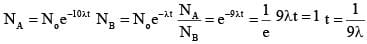JEE Main 2019 April 10 Shift 1 Paper & Solutions - Question 10

In the  given circuit,an ideal voltmeter connected  across the 10 Ω resistance reads 2V. The internal resistance r, of each cell is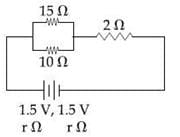Detailed Solution for JEE Main 2019 April 10 Shift 1 Paper & Solutions - Question 10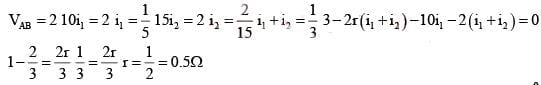JEE Main 2019 April 10 Shift 1 Paper & Solutions - Question 11

A ray of light AO in vacuum is incident on a glass slab at angle 600 and refracted at angle 300 along OB as shown in the figure.The optical path length of light ray from A to B is:

Detailed Solution for JEE Main 2019 April 10 Shift 1 Paper & Solutions - Question 11

Optical length=AO+OB A/90 =sin300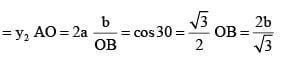.OA+OB=2a + 2b/√3

JEE Main 2019 April 10 Shift 1 Paper & Solutions - Question 12

A current of 5A passes through a copper conductor (resistivity= 1.7 ´10-8 Ωm) radius of cross section 5mm.Find the mobility of the charges if their drift velocity is 1.1x10-3 m/s.

Detailed Solution for JEE Main 2019 April 10 Shift 1 Paper & Solutions - Question 12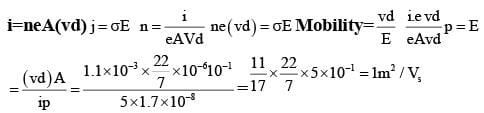JEE Main 2019 April 10 Shift 1 Paper & Solutions - Question 13

Figure shows charge (q) versus voltage (v) graph for series and parallel combination  of two given capacitors . The capacitances are :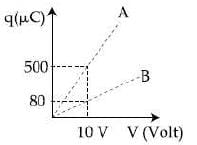JEE Main 2019 April 10 Shift 1 Paper & Solutions - Question 14

The value of acceleration due to gravity at Earth‘s surface is 9.8ms-2 . The altitude above  its surface at which the acceleration due to gravity decreases to 4.9 ms-2, is close to (Radius of earth = 6.4 x106 m. )

JEE Main 2019 April 10 Shift 1 Paper & Solutions - Question 15

Two wires A&B are carrying  currents I1 & I2 as shown in the figure . the separation between them is d . A third wire C carrying a current I is to be kept parallel to them at a distance x from A such that the net force acting on it is Zero . The possible values of x are:

Detailed Solution for JEE Main 2019 April 10 Shift 1 Paper & Solutions - Question 15

Diagram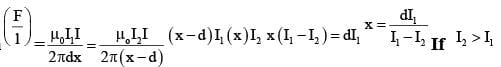Diagram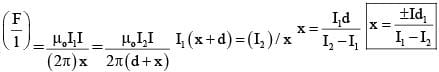JEE Main 2019 April 10 Shift 1 Paper & Solutions - Question 16

In a meter bridge experiment the circuit diagram and the corresponding observation table are shown in figure.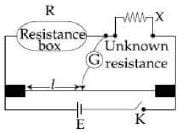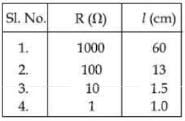Q. Which of the readings is inconsistent ?

Detailed Solution for JEE Main 2019 April 10 Shift 1 Paper & Solutions - Question 16

We know that in meter bridge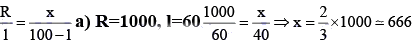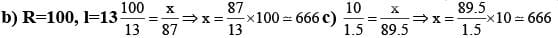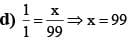Option ‘d’ is odd one So it is inconsistant.

JEE Main 2019 April 10 Shift 1 Paper & Solutions - Question 17

An npn transistor operates as a common emitter amplifier , with a power gain of 60dB. The input circuit  resistance is 100 Ω and the output load resitance is 10K Ω .The common emitter current gain β is :

Detailed Solution for JEE Main 2019 April 10 Shift 1 Paper & Solutions - Question 17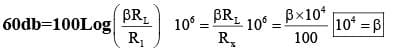JEE Main 2019 April 10 Shift 1 Paper & Solutions - Question 18

A massage signal of frequency 100MHz and peak voltage 100V is used to execute amplitude modulation on a carrier wave of frequency 300GHz and peak voltage 400 V . The modulation index and difference between the two side band frequencies are​

Detailed Solution for JEE Main 2019 April 10 Shift 1 Paper & Solutions - Question 18

Modulation index = 100v/400v = 0.25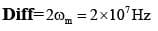JEE Main 2019 April 10 Shift 1 Paper & Solutions - Question 19

A uniformly charged ring of radius 3a and total charge q is placed in xy-plane centred at orign. A point charge q is moving towards the ring along the z-axis and has speed  v at z = 4a The minimum value of v such that it crosses the origin is:

Detailed Solution for JEE Main 2019 April 10 Shift 1 Paper & Solutions - Question 19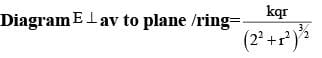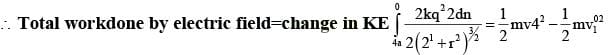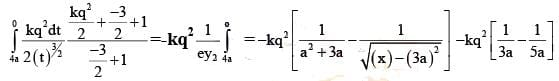JEE Main 2019 April 10 Shift 1 Paper & Solutions - Question 20

A proton,an electron, and a Helium nucleus, have the same energy. they are in circular orbits in a plane due to magnetic field perpendicular to the plane. Let rp , re and rHe be their respective radii, then,

Detailed Solution for JEE Main 2019 April 10 Shift 1 Paper & Solutions - Question 20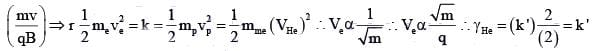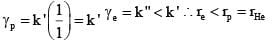JEE Main 2019 April 10 Shift 1 Paper & Solutions - Question 21

The ratio of surface tensions of mercury and water is given to be 7.5 while the ratio of their densities  is 13.6. Their contact angles, with glass, are close to 1350 and 00 respectively. it is observed that mercury gets depressed by an amount h in a capillary tube of radius r1 , while water rises by the same amount h in a capillary tube of radius r2 ,the ratio, (r1/ r2) is then close to:

Detailed Solution for JEE Main 2019 April 10 Shift 1 Paper & Solutions - Question 21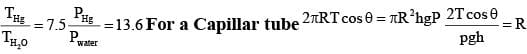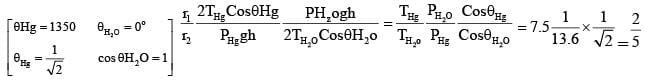JEE Main 2019 April 10 Shift 1 Paper & Solutions - Question 22

a moving Coil galvanometer allows a full scale current 10-4 A.A series resistance of2-M Ω is required  to convert the above galvanometer  into a voltmeter of range 0-5V.Therefore the value of shunt resistance  required to convert the above galvanometer in to ammeter of range 0-10mA is :

Detailed Solution for JEE Main 2019 April 10 Shift 1 Paper & Solutions - Question 22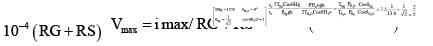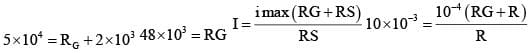JEE Main 2019 April 10 Shift 1 Paper & Solutions - Question 23

A ball is thrown upward with an initial velocity V0 from the surface of the earth. the motion of the ball is affected by a drag force equal to myV 2 ( where m is mass of the ball v is its instaneous velocity and g is a constant) . Time taken by the ball to rise to its  zenith is :

Detailed Solution for JEE Main 2019 April 10 Shift 1 Paper & Solutions - Question 23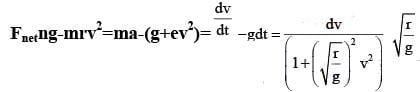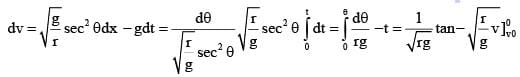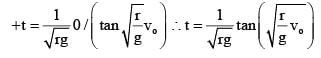JEE Main 2019 April 10 Shift 1 Paper & Solutions - Question 24

A thin disc of mass M and radius R has mass per unit area σ (r ) =kr2 where r is the distance from its center . Its moment of inertia about an axis going through its centre of mass and perpendicular to its plane is :

Detailed Solution for JEE Main 2019 April 10 Shift 1 Paper & Solutions - Question 24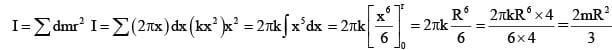JEE Main 2019 April 10 Shift 1 Paper & Solutions - Question 25

Given below in the left column are different modes of communication using the kinds of waves given in  the right column .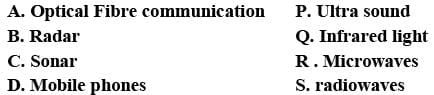From the options given below , find the most appropriate match between  entries in the left and the right column.

Detailed Solution for JEE Main 2019 April 10 Shift 1 Paper & Solutions - Question 25

Mobile phones: micro waves Mode of communication

JEE Main 2019 April 10 Shift 1 Paper & Solutions - Question 26

In an experiment , the resistance of a material is plotted as a function of temperature ( in some range) .As shown in the figure , It is a straight line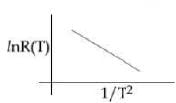Detailed Solution for JEE Main 2019 April 10 Shift 1 Paper & Solutions - Question 26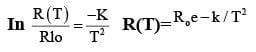JEE Main 2019 April 10 Shift 1 Paper & Solutions - Question 27

A 25 x10-3 m3 volume cylinder is field with 1 mol O2 gas room temperature (300k) The molicular diameter of O2,and its root mean square speed , are found to be 0.3 nm and 200m/s, respectively.what is the average collision rate (per second) for an O2 Molecule?

Detailed Solution for JEE Main 2019 April 10 Shift 1 Paper & Solutions - Question 27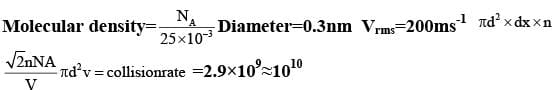JEE Main 2019 April 10 Shift 1 Paper & Solutions - Question 28

Two Coaxial discs , having moments of inertia I1and I1/2 are  rotating with respective angular velocity ω1 and ω1/2, about their  common axis.They are brought in contact with each other and thereafter they rotate with a common angular velocity . If Ef and Ei `, are the final and initial total energies,then ( Ef - Ei ) is

Detailed Solution for JEE Main 2019 April 10 Shift 1 Paper & Solutions - Question 28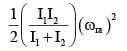JEE Main 2019 April 10 Shift 1 Paper & Solutions - Question 29

Two Particles , of masses M and 2M moving , as shown,with speeds of 10m/s and 5m/s , collied elastically at the origin. After the collision , they move along the indicated directions  with speeds  v1 and v2 ,respectively , The value of v1 and v2 are nearly: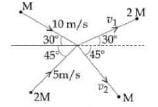Detailed Solution for JEE Main 2019 April 10 Shift 1 Paper & Solutions - Question 29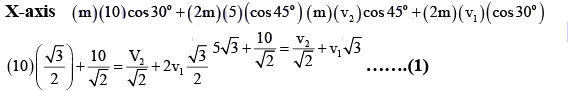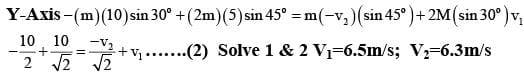JEE Main 2019 April 10 Shift 1 Paper & Solutions - Question 30

The displacement of a damped harmonic oscillator is given by x (t) = e-0.1t cos (10πt + φ) Here t it is in seconds.The time taken for its amplitude of vibration to drop to half of its initial value is close to:

Detailed Solution for JEE Main 2019 April 10 Shift 1 Paper & Solutions - Question 30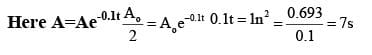JEE Main 2019 April 10 Shift 1 Paper & Solutions - Question 31

The major product of the following reaction is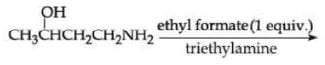Detailed Solution for JEE Main 2019 April 10 Shift 1 Paper & Solutions - Question 31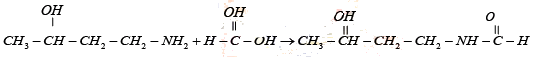JEE Main 2019 April 10 Shift 1 Paper & Solutions - Question 32

Major products of the following reaction are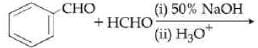Detailed Solution for JEE Main 2019 April 10 Shift 1 Paper & Solutions - Question 32

Aldehydes locking α – Hydrogen give cross cannizaro reaction
HCHO acts as hydride donor because it gives less sterically hindered tetrahedral Intermediat

JEE Main 2019 April 10 Shift 1 Paper & Solutions - Question 33

Which of the following is a condensation polymer?

Detailed Solution for JEE Main 2019 April 10 Shift 1 Paper & Solutions - Question 33

Nylon 6,6,- is a conclusion polymer of NH2 - (CH2 )6 - NH2 & Adinic Acid

JEE Main 2019 April 10 Shift 1 Paper & Solutions - Question 34

Ethylamine ( C2 H5 NH2 ) can be obtained from N-ethylphthalimide on treatment  with

Detailed Solution for JEE Main 2019 April 10 Shift 1 Paper & Solutions - Question 34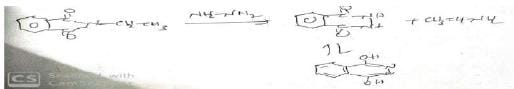## JEE Main & Advanced Mock Test Series

1 videos|356 docs|217 tests
Information about JEE Main 2019 April 10 Shift 1 Paper & Solutions Page
In this test you can find the Exam questions for JEE Main 2019 April 10 Shift 1 Paper & Solutions solved & explained in the simplest way possible. Besides giving Questions and answers for JEE Main 2019 April 10 Shift 1 Paper & Solutions, EduRev gives you an ample number of Online tests for practice

## JEE Main & Advanced Mock Test Series

1 videos|356 docs|217 tests